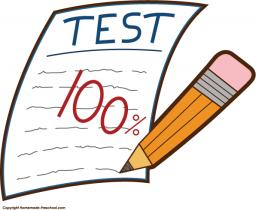# Distribution 5016

You have a test with 8 questions, where you can choose from 3 answers for each question and one answer is always correct. The probability that we answer 5 or 6 questions correctly when randomly filling in (that is, we all guess the answers) is ……. The average number of correctly guessed questions is ……. . (The quantity has a Binomial distribution of Bi and the parameters are: number of questions and probability that I will answer correctly).

p =  0.0384

### Step-by-step explanation:Did you find an error or inaccuracy? Feel free to write us. Thank you!

Tips for related online calculators
Would you like to compute the count of combinations?